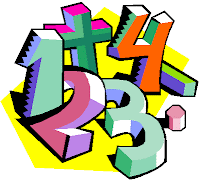# Math Puzzles

Top 10 Math PuzzlesMath Puzzles 1 : Brain Twister Puzzle
Difficulty ★★★★☆     Popularity ★★★★★

2+3=8,
3+7=27,
4+5=32,
5+8=60,
6+7=72,
7+8=??

Solve it?

=================================================

Math Puzzles 2 : Weighing Balance Puzzle
Difficulty ★★★★☆     Popularity ★★★★☆

You can place weights on both side of weighing balance and you need to measure all weights between 1 and 1000. For example if you have weights 1 and 3,now you can measure 1,3 and 4 like earlier case, and also you can measure 2,by placing 3 on one side and 1 on the side which contain the substance to be weighed. So question again is how many minimum weights and of what denominations you need to measure all weights from 1kg to 1000kg.

=================================================

Math Puzzles 3 : Gold Bar Fewest Cut Puzzle
Difficulty ★★★☆☆     Popularity ★★★★☆

A worker is to perform work for you for seven straight days. In return for his work, you will pay him 1/7th of a bar of gold per day. The worker requires a daily payment of 1/7th of the bar of gold. What and where are the fewest number of cuts to the bar of gold that will allow you to pay him 1/7th each day?

=================================================

Math Puzzles 4 : Secret Code puzzle
Difficulty ★★★☆☆     Popularity ★★★★☆

A man wanted to get into his work building, but he had forgotten his code. However, he did remember five clues. These are what those clues were:

The fifth number plus the third number equals fourteen.

The fourth number is one more than the second number.

The first number is one less than twice the second number.

The second number plus the third number equals ten.

The sum of all five numbers is 30.

What were the five numbers and in what order?

=================================================

Math Puzzles 5 : Simple Simple Google Interview Puzzle
Difficulty ★★☆☆☆     Popularity ★★★☆☆

The puzzle is if the shopkeeper can only place the weights in one side of the common balance. For example if shopkeeper has weights 1 and 3 then he can measure 1, 3 and 4 only. Now the question is how many minimum weights and names the weights you will need to measure all weights from 1 to 1000. This is a fairly simple problem and very easy to prove also.  Answer for this puzzle is given below.

=================================================

Math Puzzles 6 : CAT Exam Puzzle
Difficulty ★★★☆☆     Popularity ★★★☆☆

5+3+2 = 151022
9+2+4 = 183652
8+6+3 = 482466
5+4+5 = 202541
THEN ;
7+2+5 =

=================================================

Math Puzzles 7 : Interesting Number puzzle
Difficulty ★★★☆☆     Popularity ★★★☆☆

A number with an interesting property:

When I divide it by 2, the remainder is 1.
When I divide it by 3, the remainder is 2.
When I divide it by 4, the remainder is 3.
When I divide it by 5, the remainder is 4.
When I divide it by 6, the remainder is 5.
When I divide it by 7, the remainder is 6.
When I divide it by 8, the remainder is 7.
When I divide it by 9, the remainder is 8.
When I divide it by 10, the remainder is 9.

It's not a small number, but it's not really big, either.
When I looked for a smaller number with this property I couldn't find one.

Can you find it?

=================================================

Math Puzzles 8 : Distance Puzzle
Difficulty ★★☆☆☆     Popularity ★★☆☆☆

Two friends decide to get together; so they start riding bikes towards each other. They plan to meet halfway. Each is riding at 6 MPH. They live 36 miles apart. One of them has a pet carrier pigeon and it starts flying the instant the friends start traveling. The pigeon flies back and forth at 18 MPH between the 2 friends until the friends meet.

How many miles does the pigeon travel?

=================================================

Math Puzzles 9 : Train Puzzle
Difficulty ★★☆☆☆     Popularity ★★☆☆☆

Charles walks over a railway-bridge. At the moment that he is just ten meters away from the middle of the bridge, he hears a train coming from behind. At that moment, the train, which travels at a speed of 90 km/h, is exactly as far away from the bridge as the bridge measures in length. Without hesitation, Charles rushes straight towards the train to get off the bridge. In this way, he misses the train by just four meters! If Charles would, however, have rushed exactly as fast in the other direction, the train would have hit him eight meters before the end of the bridge.

What is the length of the railway-bridge?

=================================================

Math Puzzles 10 : Hardest Mathematical Columbus Puzzle
Difficulty ★★★★★     Popularity ★★☆☆☆

The Puzzle: Here is a famous prize problem that Sam Loyd issued in 1882, offering \$1000 as a prize for the best answer showing how to arrange the seven figures and the eight 'dots' .4.5.6.7.8.9.0. which would add up to 82